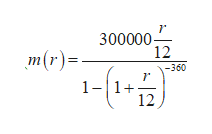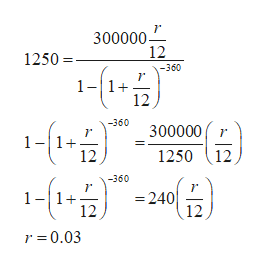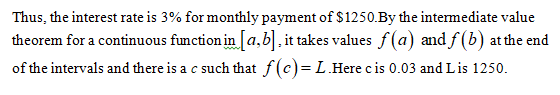After getting a great job from your prestigious CSM degree, you are shopping for a \$300,000, 30-year home loan.The monthly payment is m(r) = (300000 r /12) / 1 − (1 + r 12 ) −360,where r is the annual interest rate. Current interest rates are anywhere between 2% (r = 0.02) and 5% (r = 0.05), depending on credit.(a) Use the Intermediate Value Theorem to show that there is an interest rate between 2 and 5 percent that allows you to make a monthly payment of \$1250. Show that all conditions of the theorem are met and correctly state the conclusion in context. Be specific about the intervals for this problem; don’t just state IVT generally.

Question

After getting a great job from your prestigious CSM degree, you are shopping for a \$300,000, 30-year home loan.

The monthly payment is m(r) = (300000 r /12) / 1 − (1 + r 12 ) −360

,where r is the annual interest rate. Current interest rates are anywhere between 2% (r = 0.02) and 5% (r = 0.05), depending on credit.

(a) Use the Intermediate Value Theorem to show that there is an interest rate between 2 and 5 percent that allows you to make a monthly payment of \$1250. Show that all conditions of the theorem are met and correctly state the conclusion in context. Be specific about the intervals for this problem; don’t just state IVT generally.

Step 1

Given that the monthly payment ishelp_outlineImage Transcriptionclose300000 12 -360 m(r) 1 -1 12 fullscreen
Step 2

The interest rate r must lie between 0.02 and 0.05 for a monthly payment of \$1250.help_outlineImage Transcriptionclose300000스 12 1250 -360 1-1+ 12 -360 300000 1-1+ 12 125012 -360 1-1+ =240 12 12 r 0.03 fullscreen
Step 3...

Want to see the full answer?

See Solution

Want to see this answer and more?

Our solutions are written by experts, many with advanced degrees, and available 24/7

See Solution
Tagged in

Other